# Draw Shapes

Draw rectangles, lines, polygons, or circles on images

• Library:
• Computer Vision Toolbox / Text & Graphics

•## Description

The Draw Shapes block draws rectangles, lines, polygons, or circles on images by overwriting pixel values. As a result, the shapes are embedded in the output image. The block uses Bresenham's line drawing algorithm to draw lines, polygons, and rectangles and Bresenham's circle drawing algorithm to draw circles. The output signal is the same size and data type as the inputs to the Image, R, G, and B ports.

You can set the shape fill or border color via the input port or by manually entering the values in the Block Parameters window. Use the color parameters to determine the appearance of the rectangles, lines, polygons, or circles.

## Ports

### Input

expand all

Input image, specified as either an M-by-N matrix of intensity values or an M-by-N-by-P matrix of color values with P color planes.

Data Types: `single` | `double` | `int8` | `int16` | `int32` | `int64` | `uint8` | `uint16` | `uint32` | `uint64` | `Boolean` | `fixed point`

Red video stream, specified as a scalar, vector, or a matrix. The input represents one plane of the RGB video stream. The R, G, and B ports must have the same dimensions and data type.

#### Dependencies

To enable this port, set the Image signal parameter to `Separate color signals`.

Data Types: `single` | `double` | `int8` | `int16` | `int32` | `int64` | `uint8` | `uint16` | `uint32` | `uint64` | `Boolean` | `fixed point`

Green video stream, specified as a scalar, vector, or a matrix. The input represents one plane of the RGB video stream. The R, G, and B ports must have the same dimensions and data type.

#### Dependencies

To enable this port, set the Image signal parameter to `Separate color signals`.

Data Types: `single` | `double` | `int8` | `int16` | `int32` | `int64` | `uint8` | `uint16` | `uint32` | `uint64` | `Boolean` | `fixed point`

Blue video stream, specified as a scalar, vector, or a matrix. The input represents one plane of the RGB video stream. The R, G, and B ports must have the same dimensions and data type.

#### Dependencies

To enable this port, set the Image signal parameter to `Separate color signals`.

Data Types: `single` | `double` | `int8` | `int16` | `int32` | `int64` | `uint8` | `uint16` | `uint32` | `uint64` | `Boolean` | `fixed point`

Shape coordinates. The format of the points depends on the type of shape you specify with the Shape parameter.

• `Rectangles`M-by-4 matrix of M rectangles. The format for each rectangle must be, [x y width height].

ShapeInput to the Pts PortDrawn Shape
Single RectangleFour-element row vector in the format
`[x y width height]`, where:
• `x` and `y` are the one-based coordinates of the upper-left corner of the rectangle.

• `width` and `height` are the width and height in pixels, of the rectangle. The values of `width` and `height` must be greater than 0.M Rectangles

M-by-4 matrix in the format

`$\left[\begin{array}{cccc}{x}_{1}& {y}_{1}& widt{h}_{1}& heigh{t}_{1}\\ {x}_{2}& {y}_{2}& widt{h}_{2}& heigh{t}_{2}\\ ⋮& ⋮& ⋮& ⋮\\ {x}_{M}& {y}_{M}& widt{h}_{M}& heigh{t}_{M}\end{array}\right]$`

,where each row of the matrix corresponds to a different rectangle and is of the same form as the vector for a single rectangle.• `Lines`M-by-2L matrix of M polylines. The format for each polyline must be of the form [x1,y1,x2,y2,…,xL,yL].

ShapeInput to the Pts PortDrawn Shape
Single LineFour-element row vector in the format ```[x1 y1 x2 y2]```, where:
• `x1` and `y1` are the coordinates of the beginning of the line.

• `x2` and `y2` are the coordinates of the end of the line.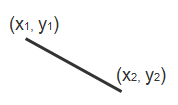M Lines

M-by-4 matrix in the format

`$\left[\begin{array}{cccc}{x}_{11}& {y}_{11}& {x}_{12}& {y}_{12}\\ {x}_{21}& {y}_{21}& {x}_{22}& {y}_{22}\\ ⋮& ⋮& ⋮& ⋮\\ {x}_{M1}& {y}_{M1}& {x}_{M2}& {y}_{M2}\end{array}\right]$`

,where each row of the matrix corresponds to a different line and is of the same form as the vector for a single line.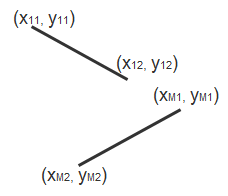Single Polyline with (L-1) SegmentsVector of size 2L, where L is the number of vertices, in the format, ```[x1, y1, x2, y2, ..., xL, yL```, where
• x1 and y1 are the coordinates of the beginning of the first line segment.

• x2 and y2 are the coordinates of the end of the first line segment and the beginning of the second line segment.

• xL and yL are the coordinates of the end of the (L-1)th line segment.

The polyline always contains (L-1) number of segments because the first and last vertex points do not connect. The block produces an error message when the number of rows is less than two or not a multiple of two.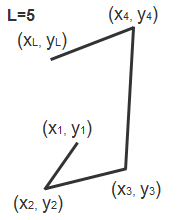M Polylines with (L-1) Segments

M-by-2L matrix in the format

`$\left[\begin{array}{ccccccc}{x}_{11}& {y}_{11}& {x}_{12}& {y}_{12}& \cdots & {x}_{1L}& {y}_{1L}\\ {x}_{21}& {y}_{21}& {x}_{22}& {y}_{22}& \cdots & {x}_{2L}& {y}_{2L}\\ ⋮& ⋮& ⋮& ⋮& \ddots & ⋮& ⋮\\ {x}_{M1}& {y}_{M1}& {x}_{M2}& {y}_{M2}& \cdots & {x}_{ML}& {y}_{ML}\end{array}\right]$`

, where each row of the matrix corresponds to a different polyline and is of the same form as the vector for a single polyline. When you require one polyline to contain less than (L–1) number of segments, fill the matrix by repeating the coordinates of the last vertex.

The block produces an error message if the number of rows is less than two or not a multiple of two.• `Polygons`M-by-2L matrix of M polygons. The format for each polygon must be of the form [x1,y1,x2,y2,…,xL,yL]. The points are specified in consecutive order to form a polygon.

ShapeInput to the Pts PortDrawn Shape
Single Polygon with L line segmentsRow vector of size 2L, where L is the number of vertices, in the format ```[x1 y1 x2 y2 ... xL yL]```, where:
• x1 and y1 are the coordinates of the beginning of the first line segment.

• x2 and y2 are the coordinates of the end of the first line segment and the beginning of the second line segment.

• xL and yL are the coordinates of the end of the (L-1)th line segment and the beginning of the Lth line segment.

The block connects ```[x1 y1]``` to ```[xL yL]``` to complete the polygon. The block produces an error if the number of rows is negative or not a multiple of two.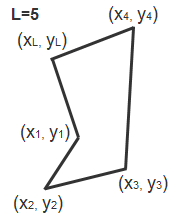M Polygons with the largest number of line segments in any line being L

M-by-2L matrix in the format

`$\left[\begin{array}{ccccccc}{x}_{11}& {y}_{11}& {x}_{12}& {y}_{12}& \cdots & {x}_{1L}& {y}_{1L}\\ {x}_{21}& {y}_{21}& {x}_{22}& {y}_{22}& \cdots & {x}_{2L}& {y}_{2L}\\ ⋮& ⋮& ⋮& ⋮& \ddots & ⋮& ⋮\\ {x}_{M1}& {y}_{M1}& {x}_{M2}& {y}_{M2}& \cdots & {x}_{ML}& {y}_{ML}\end{array}\right]$`

, where each row of the matrix corresponds to a different polygon and is of the same form as the vector for a single polygon. If some polygons are shorter than others, repeat the ending coordinates to fill the polygon matrix.

The block produces an error message if the number of rows is less than two or is not a multiple of two.• `Circles`M-by-3 matrix of M circles. The format for each circle must be, [x y radius].

ShapeInput to the Pts PortDrawn Shape
Single CircleThree-element row vector in the format
`[x y radius]`, where:
• `x` and `y` are coordinates for the center of the circle.

• `radius` is the radius of the circle, which must be greater than 0.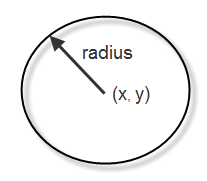M Circles

M-by-3 matrix in the format

`$\left[\begin{array}{ccc}{x}_{1}& {y}_{1}& radiu{s}_{1}\\ {x}_{2}& {y}_{2}& radiu{s}_{2}\\ ⋮& ⋮& ⋮\\ {x}_{M}& {y}_{M}& radiu{s}_{M}\end{array}\right]$`

, where each row of the matrix corresponds to a different circle and is of the same form as the vector for a single circle.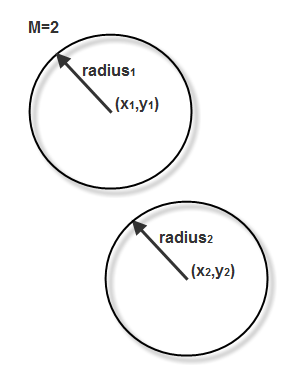#### Dependencies

• Double-precision floating point is only supported if the input to the Image or R, G, and B ports is floating point.

• Single-precision floating point (only supported if the input to the Image or R, G, and B ports is floating point).

Data Types: `single` | `double` | `int8` | `int16` | `int32` | `uint8` | `uint16` | `uint32`

Region of interest, specified as a four-element vector [x y width height]. The region defines a rectangular area to draw shapes. The first two elements represent the one-based coordinates of the upper-left corner of the area. The second two elements represent the width and height of the area.

#### Dependencies

To enable this port, set the ROI port appears when you set the Draw shapes in parameter to ```Specify region of interest via port```.

Data Types: `single` | `double` | `int8` | `int16` | `int32` | `int64` | `uint8` | `uint16` | `uint32` | `uint64` | `Boolean` | `fixed point`

Color of shape, specified as either a P-element vector or an M-by-P matrix of M number of shapes and P number of color planes. You can specify a color (RGB) for each shape, or specify one color for all shapes.

#### Dependencies

To enable this port, set the Border color source parameter to Input port.

Data Types: `single` | `double` | `int8` | `int16` | `int32` | `int64` | `uint8` | `uint16` | `uint32` | `uint64` | `Boolean` | `fixed point`

### Output

expand all

Output image, returned as a scalar, vector, or matrix.

Data Types: `single` | `double` | `int8` | `int16` | `int32` | `int64` | `uint8` | `uint16` | `uint32` | `uint64` | `Boolean` | `fixed point` | `enumerated` | `bus`

## Parameters

expand all

Main Tab

Select Fill Shapes to specify an intensity value or a color.

Note

If you are generating code and you select the Fill shapes check box, the word length of the block input(s) cannot exceed 16 bits.

Specify the source for the fill color value to either ```Specify via dialog``` or `Input port`.

#### Dependencies

To enable this parameter, select Fill shapes.

Specify the shape fill color.

#### Dependencies

To enable this parameter, select the Fill shapes parameter.

Specify the source for the border color value to either ```Specify via dialog``` or `Input port`.

#### Dependencies

To enable this parameter, select the Fill shapes parameter.

Specify the appearance of the shape's border. You can specify `Black`, `White`, or `User-specified value`. This table describes what to enter for the color value based on the block input and the number of shapes you are drawing.

Block InputColor Value(s) for Drawing One Shape or Multiple Shapes with the Same ColorColor Value(s) for Drawing Multiple Shapes with Unique Colors
Intensity imageScalar intensity valueR-element vector where R is the number of shapes
Color imageP-element vector where P is the number of color planesR-by-P matrix where P is the number of color planes and R is the number of shapes

For each value in the Color Value(s) parameter, enter a number between the minimum and maximum values that can be represented by the data type of the input image. If you enter a value outside this range, the block produces an error message.

#### Dependencies

To enable this parameter, clear the Fill shapes parameter.

Specify an intensity or color value for the shape's border or fill.

Tunable: Yes

#### Dependencies

To enable this parameter set the Border color or Fill color parameter to ```User-specified value```.

Specify the opacity of the shading inside the shape, where `0` is transparent and `1` is opaque.

Opacity Factor value for Drawing One Shape or Multiple Shapes with the Same ColorOpacity Factor value for Drawing Multiple Shapes with Unique Colors
Scalar intensity valueR-element vector where R is the number of shapes

#### Dependencies

To enable this parameter, clear the Fill shapes parameter.

Perform a smoothing algorithm on the line, polygon, or circle.

#### Dependencies

• To enable this parameter, set the Shape parameter to either `Lines`, `Polygons`, or `Circles`.

Specify how to input and output a color video signal. Select the color video signal as one of the following:

• `One multidimensional signal` — The block accepts an M-by-N-by-P color video signal, where P is the number of color planes, at one port.

• `Separate color signals` — Enables the R,G, and B input ports. Each port accepts one M-by-N plane of an RGB video stream.

Data Types Tab

For details on the fixed-point block parameters, see Specify Fixed-Point Attributes for Blocks.

## Block Characteristics

 Data Types `Boolean` | `double` | `fixed point` | `integer` | `single` Multidimensional Signals `yes` Variable-Size Signals `yes`

## Version History

Introduced before R2006a